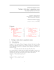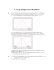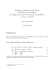# Stat 100 Sample Final Questions

## Transcription

Stat 100 Sample Final Questions
```Stat 100 Sample Final Questions
1. Suppose the ages of 19 children at a day care children are as follows (in months):
36, 42, 18, 32, 22, 22, 25, 29, 31, 19, 30, 24, 35, 29, 26, 36, 24, 28, 28
a) Classify the data as (e.g. quantitative/continuous)
b) Find the mean, variance and standard deviation of this sample
c) Find the Five Number Summary and the interquartile range.
d) Based on the Five Number Summary, what term best describes the shape of the
distribution?
e) Using the method based on Q3 and the IQR, would you consider the oldest child to be
an “outlier”?
2. Consider the data on number of minor and serious work-related accidents at a factory, broken
up by male and female employees:
Minor Accident
Major Accident
TOTAL
Male Worker
34
12
46
Female Worker
13
8
21
TOTAL
47
20
67
What is the probability that a randomly selected accident at this factory ...
a) ...happened to a female worker?
b) ...was a major accident?
c) ...was a minor accident by a male worker?
d) ...was a major accident, given that we know it happened to a male worker?
e) ...happened to a male worker, given that we know it was major?
Are the events “accident was major” and “accident happened to a female worker”...
f)... independent?
g)...mutually exclusive?
3. Find the given probabilities:
a) Two fair dice are thrown. Find the probability that their sum is at least ten.
b) Two cards are drawn without replacement from a standard 52-card deck. What is the
probability that at least one is a heart?
c) A coin is flipped three times. What is the probability heads comes up more often than
tails?
4. It is known that one out of ten men cannot distinguish between the colours red and green. This
type of colour blindness causes problems with traffic signals.
a) If six men are randomly selected for a study of traffic signal perceptions, find the
probability that at least two of them cannot distinguish between red and green.
b) In a major study of 600 men, find the probability that at least 50 cannot distinguish
between red and green.
5. Suppose the respective probabilities are 0.7, 0.2, and 0.1 that a person applying for a driver’s
licence in Saskatchewan will require 1, 2, or 3 attempts in order to obtain a licence. Let X
be a random variable, the number of attempts in order to obtain a licence. Find the mean
(expected value) of X.
6. The cholesterol content of large eggs of a particular brand is normally distributed with a mean
of 200 milligrams and standard deviation 15 milligrams.
a) What proportion of these eggs have cholesterol content above 205 milligrams?
b) What is the probability that the mean cholesterol content of a random sample of 25 of these
eggs is less than 205 milligrams?
c) In sixty-seven percent of the eggs, the cholesterol content is less than a certain value “v”.
Find the value of “v”.
7. A political candidate estimates that 30% of all voters support her proposed tax reform bill.
a) Assuming her estimate is correct and there are 400 voters at a rally , find the probability that
at most 25% (that is, at most 100) favour her tax bill.
b) If you observed that 100 of the 400 voters at the rally supported her tax bill, would you
conclude that her estimate of 30% is too high? (perform an appropriate hypothesis test,
using a 5% level of significance)
8. A random sample of 5 mechanics took 5, 8, 12, 14, and 16 minutes to assemble a certain
device. Assume the population of all such assembly times is normally distributed.
a) What is the point estimate for the true mean assembly time for this device?
b) Construct a 95% confidence interval for the true mean time to assemble this device.
c) At the 5% level of significance, would you reject the claim that the mean of the population
of all such assembly times is 15 minutes?
d) Suppose it is later decided that the population standard deviation is F=/20. Would this
change your conclusion in part (b)?
9. If you wanted to estimate the true percentage of all voters in Regina who are in favour of
abolition of the senate, and if you wanted your maximum error of estimate to be 6% with
a confidence level of 95%, what would the be the required sample size?
10. If you wanted to estimate the true mean temperature of Grande Latte’s served at a local
starbucks, how many Grande Latte’s would you need to sample to have an estimate with a
maximum error of 30 C, 9 out of 10 times? You may assume that F = 130 C.
11. The manager of a shipping company believes the average truckload delivers 2500 kg of
goods. A shareholder argues that this is an inflated figure to lure new investors. (S)he
randomly samples the records of 25 loads and finds the mean to be 2460 kg with a
standard deviation of 250 kg. Can the manager’s claim be rejected using "=0.05?
12. A bottling machine is said to be operating properly if it produces bottles that are not full in at
most 5% of all cases. A random sample of 100 bottles had 7 bottles that were not full.
Using a significance level of 0.01, test the claim that the machine is operating properly.
Calculate the P-value.
13. A researcher wishes to establish a connection between sleep deprivation and physical
strength. 16 subjects are asked to perform as many bench presses as possible, then are
asked to stay awake for 24 hours, and repeat the experiment. The following table shows
the number of bench presses performed before and after the sleep deprivation for each
subject:
Subj
1
2
3
4
5
6
7
8
9
10
11
12
13
14
15
16
Pre
1
9
11
9
51
60
23
20
32
16
11
26
45
26
10
8
15
Post
1
9
10
8
51
58
21
17
28
16
9
22
40
20
7
6
11
Is there enough evidence ("=0.01) to show that sleep deprivation decreases the number of
bench presses?
14. A store manager advertises that at least 90% of their customers are satisfied. A consumer
activist wishes to put this claim to the test and randomly polls 150 of the store’s
customers. 132 said they were satisfied. Find the P-value and use it to discuss the
significance of this result.
15. The discharge of industrial wastewater into rivers affects water quality. To assess the effect of
a particular power plant on water quality, 60 water specimens are taken 16km upstream
from the plant and 60 water specimens were taken 4 km downstream from the plant.
Alkalinity (mg/l) was determined for each specimen, resulting in the summary quantities
below.
Location
Upstream
Downstream
n
60
60
Mean
84.2
91.6
Standard Deviation
19.4
18.8
a) At the 0.01 level of significance, does the data suggest that the true mean alkalinity is higher
downstream than upstream?
b) Find the P-value corresponding to the observed value of your test statistic in (a) above.
c) Suppose the significance level is changed from 0.01 to 0.04. Use the P-value found in part
(b) to decide whether your answer in part (a) would remain the same.
16. In a sample of 100 store customers, 43% used a MasterCard. In another sample of 100, 58%
used a Visa card. Construct a 98% confidence interval estimate for the difference between
the true percentage of all customers who use a MasterCard and the true percentage of all
customers who use a Visa card.
17. Two paired variables X and Y have linear correlation coefficient r=-0.82 and best fit
regression line
.
a) What does the value of r tell you?
b) What response Y does this model predict when X=15?
18. The given table lists both the Caribou (X) and Wolf (Y) populations at a national park over
the past seven years. (Caribou populations are listed in hundreds of animals).
Caribou
30
34
27
25
17
23
20
Wolf
66
79
70
60
48
55
60
a) Calculate the correlation coefficient.
b) A student claims that the linear regression line has equation Y=21.5 - 3.5 X.
Without any calculations, how do you know (s)he is incorrect?
c) Calculate the correct equation of the regression line.
d) In your own words, what does the slope “b” represent in this case?
e) What does the model predict about the Wolf population if there are 24 (hundred)
Caribou?
f) What does the model predict about the Wolf population if there are no Caribou. Would
you trust this value? Why or why not?
1.
a)
This data is quantitative, discrete.
b)
First, find n=19, 3x=536 and 3x2 = 15822.
Hence
mean 0=3x/n = 28.21 months,
variance s2 = [3x2 - (3x)2/n] / (n-1) = 38.95
and standard deviation s=6.24 months.
c)
First, we need to list the data in order:
18, 19, 22, 22, 24, 24, 25, 26, 28, 28, 29, 29, 30, 31, 32, 35, 36, 36, 42
With 19 data points, the first quartile is at position (0.25)(n+1)=5. The median is at
position (0.5)(n+1)=10. The third quartile is at position (0.75)(n+1)=15.
Hence the Five number summary is:
Min = 18
Q1=24
Median=28
Q3=32
Max=42
The inter-quartile range is IQR=Q3-Q1=8
d)
Based on the five number summary, the data has a slight positive skew (note that the Max
is further from the median than the Min).
e)
Using the Q3 and the IQR, the boundary for an outlier is
Q3+(1.5)(IQR) = 44 months.
Hence the oldest (at 42 months) is not considered an outlier.
2.
a)
b)
c)
d)
e)
P(female)=21/67
P(major)=20/67
P(minor AND male)=34/67
P(major | male) = 12/46
P(male | major) = 12/20
f)
To test for independence we must check P(female)=P(female|major) (or vice versa)
P(female)=21/67 . 0.31
P(female|major)=8/20 = 0.4
These probabilities are not equal, hence the events are dependent.
g)
The events are not mutually exclusive, since we can have an overlap (accidents that
happened to female workers).
3.
a) Possibilities are {46, 55, 64, 56, 65, 66}. Hence the probability is P(E)=6/36 = 1/6
b) Do negation: the probability of picking no heart is P(no heart)=(39/52) (38/51)
Hence the probability of at least one heart is
P(at least one heart)= 1 - P(no heart) . 1- 0.5588 = 0.4412
c) This is binomial with n=3, p=0.5, q=0.5. Let X be the number of times heads comes
up. We need P(X\$2) = P(X=2) + P(X=3)
= (3) (0.5)2(0.5) + (0.5)3 = 0.5.
As expected, the probability is ½.
4. It is known that one out of ten men cannot distinguish between the colours red and green. This
type of colour blindness causes problems with traffic signals.
a) If six men are randomly selected for a study of traffic signal perceptions, find the
probability that at least two of them cannot distinguish between red and green.
b) In a major study of 600 men, find the probability that at least 50 cannot distinguish
between red and green.
4.
a) Let X be # of colourblind men. Use binomial probability with n=6, p=.1, q=.9
P(X\$2) = 1 - P(X=0) - P(X=1) = 1 - .53144 - .35429 = .11427
b) Use the normal approximation with n=600, p=.1, q=.9.
We have mean :=np=60 and standard deviation F=/(npq)=7.35
Find the probability P(X>49.5).
Convert x=49.5 to z=-1.43.
Hence P(z<-1.43)=0.0764.
The probability of having at least 50 such men is 1-0.0764 = .9236
5. Simple calculation, expected value :=E(X)=3xP(x) = 1.4. On average it will take people 1.4
6. a) P(x>205) = P(z>.33) = .3707
b) Sampling distribution! Convert using z=(0-:)/(F//n)
P(0<205) = P(z<1.67) = .9525
c) We are looking for v, such that P(x<v)=.67
Look up A=.67 to find z=.44, then solve for x=:+zF=206.6
Hence the value of “v” is 206.6 mg.
7. a) This is a binomial distribution, n=400, p=.3, q=.7. Use the normal approximation to find:
P(p#25%) = P(x<100.5) = P(z<-2.13) = .0166
The probability is .0166.
b)
Hypothesis:
H0: p\$0.30
H1:p<0.30 (claim)
Rejection region: Large sample proportion test, use z-scores.
Rejection region for a one-tailed test with "=0.05 is z<-1.645.
Test statistic
Decision:
Reject H0.
At "=0.05, there is sufficient evidence that the 30% estimate is too high.
8. a) The point estimate is simply the sample mean, i.e. :.0 =11 minutes.
b) Work out 0=11 and s=/20, use t-values (d.f.=4) and we must assume (already stated,
though) that the population assembly times are normally distributed.
The t-value is t=2.776. This gives a maximum error of estimate E=(2.776) /20//5
= 2.48 minutes
So the CI is
or
0 - E< : < 0 + E
5.45 < : < 16.55 minutes.
c) Note that we have a two tailed test with "=0.05 -> equivalent to doing a 95% conidence
interval. Since the hypothesised value of :=15 minutes lies in the confidence interval, we
fail to reject H0: :=15 minutes. There is no statistically significant evidence to reject that
the true mean assembly time is 15 minutes.
d) If F is known we can use z-values rather than t-values. The rejection region changes to
z<-1.96 or z>1.96. Now we need to calculate our test statistic as z=-2. Our test statistic is
in the rejection region, and we would reject H0. Using this additional piece of
information, our decision would change to reject the claim that the assembly time is 15
minutes.
9. Solve E = z.025 /[pq/n] , with E=.06 and z0.25=1.96. Since no previous data on p is given,
me must assume the most conservative estimate, i.e. p=.5 and q=.5. Solve this for
n=266.7. Hence sample n=267 voters.
10. Solve E= z F / /n
, with E=3 and z=1.645,
for n=(zF/E)2 = 50.8.
You need to sample 51 Grande Latte’s. Just don’t drink them all at once.
11.
Hypothesis:
H0: :\$2500 kg.
H1: :<2500 kg (claim)
Rejection Region: Small sample, must use t-scores (and must assume population is
normal) with d.f.=24. With "=0.05 we will reject if t<-1.711.
Test Statistic: Calculate t= (2460-2500) / (250//25) = -.80.
Decision: Do not reject H0.
Answer: At "=0.05, there is insufficient evidence to doubt the manager’s claim.
12.
Hypothesis:
H0: p#0.05 (claim)
H1: P>0.05
Rejection Region: "=0.01, one sided, critical value is z=2.326
Test-Statistic: Use z-scores (large sample proportion).
Calculate our z=(.07-.05)/.0218 = .92.
Decision: We do not reject H0.
Answer: At "=0.01, there is no evidence to reject the hypothesis that the machine is
working properly.
P-Value: The probability beyond z=.92 (one tail) is P=.5 - .3212 = .1788.
13. This is a paired t-test, i.e. we are using two small dependent samples.
Let new variable D=pre-post.
Hypothesis:
H0: :D#0.
H1: :D>0 (claim)
Rejection Region: Using t-scores with d.f.=15 we get a critical value of t=2.602
Test Statistic: First compute (from the 16 differences)
D(bar)=2.437 and sD=1.825.
Then compute a t-value of t= (D(bar) - 0) / sD = 5.34.
Decision: We reject H0.
Answer: At "=0.01, there is sufficient evidence that sleep deprivation does cause a
decrease in the true mean number of bench presses.
14.
Hypothesis:
H0: p\$.9 (claim)
H1: p<.9.
Test-Statistic: Use z-scores. Calculate our z-score to be z=-.83
P-Value: The area beyond -.83 is P= .2033.
Decision: This is a vary high P-value, hence these results are not very significant at all. In
other words, we have little reason to doubt the advertised claim based on our sample.
15.a) Let Population 1 be upstream, Population 2 be downstream.
This is a hypothesis test for two population means (independent, large samples).
H0: :1\$:2
H1: :1<:2 (claim)
Rejection Region: We are using two large samples, hence approximating via z-scores.
The critical value for a one-tailed test with "= 0.01 is z=-2.326.
Hypothesis:
Test Statistic: 01-02 = -7.4,
:1-:2=0, and standard error F01-02 = 3.49, gives a test statistic of z=-2.12.
Decision: Do not reject H0.
Answer: At "=0.01, the data does not suggest a higher alkalinity level downstream.
b) P-Value is P(z<-2.12) = .017
c) Yes, our answer would change, as our P-value is now less than the new significance level
0.04. We would now reject H0 and conclude that there is sufficient evidence of higher
alkalinity downstream.
16. This is a confidence interval for two population proportions. We use z.01=2.326 for this
interval, and can calculate p1(hat)-p2(hat)=-0.15 and standard error
,
Hence the maximum error of the estimate is E= (2.326)(.07)
and the confidence interval is -0.15 - 2.326 * .07 < p1-p2 < -0.15 + 2.326 * .07,
Hence the 98% confidence interval estimate for the difference in proportion of customers
using MasterCard and Visa is
-0.313 <p1-p2< 0.0128
17.
a)
b)
The correlation coefficient indicates that the regression line will fit the data fairly well,
and that the line will have a negative slope.
The expected response is Y=20.65
18.
a) The correlation coefficient is r=0.915.
b) We can see that the slope of the regression line must be positive (either by inspecting the data,
or looking the sign of r). Hence his/her result cannot be correct.
c) Calculate y-intercept a=22.35 and slope b=1.6
Hence Y(hat)=22.35 + 1.6X
d) In this case we can interpret b as the increase in the wolf population we would expect if the
Caribou population increases by 1 (hundred). In other words, 100 Caribou are expected to
e) Using X=24, we find an expected value of Y=60.75 wolves.
f) Our result would be the y-intercept, i.e. Y=22.35. There is, however, little value to this number
as X=0 lies far outside of the observed values for X. We do not know if the linear
relationship still holds at this point.
```

### Document 6537334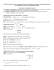### 7.6 Large-Sample Test of Hypothesis about a Population p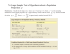### Chapter 11 and 12 Review 2015 ANSWERS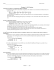### Hypothesis Testing Series (One Sample) STA2023-4a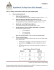### Chapter 7 Lecture Notes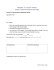### STAT 2000 Sample Midterm (actually given in October 2007)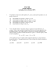### Chapter 6: Confidence Intervals and Hypothesis Testing Section 6.1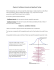### AP Statistics Name:_________________________________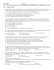### Math 116 - Final Review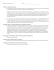### Math 119 Sample Final Exam Open book and note Calculator OK Multiple Choice  1 point each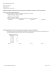### Inferential Statistics t and ScWk 242 – Session 9 Slides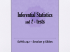### One-Sample Hypothesis Tests Review Problems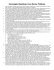### What is Hypothesis Testing? AP Statistics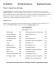### Chapter 8 practice - faculty.piercecollege.edu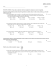### chapter 9 practice - faculty.piercecollege.edu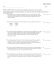### How to write a Research Grant? or Spencer Gibson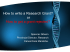### Sampling Distributions and One Sample Tests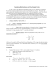### Lecture 1.2 Confidence Interval Estimation 2. Introduction to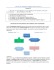### Testing a claim about a population mean